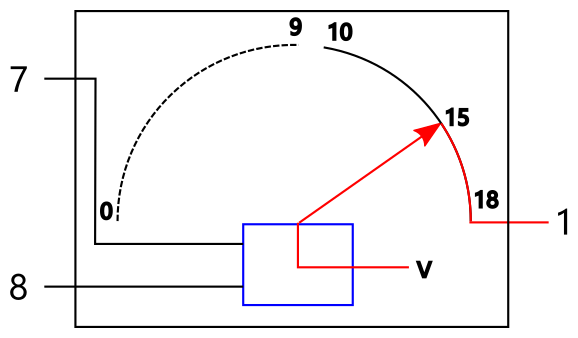## 计算机是如何做加法的？(4)——构建半加器的初步设想

### 目录## 进一步分解

S=f(A, B)

C=f(A, B)``````public Output halfAdd(Input a, Input b) {
Sum s = sum(a, b);
Carry c = carry(a, b);
return new Output(s, c);
}

public Sum sum(Input a, Input b) {
// TODO
return null;
}

public Carry carry(Input a, Input b) {
// TODO
return null;
}
``````## 进位器

0+0 –> 0

0+1 –> 0

5+4 –> 0

5+5 –> 1

9+9 –> 1## 加位器

0+0 –> 0

0+1 –> 1

5+4 –> 9

5+5 –> 0

9+9 –> 8## 二进制：A Great Insight

All the information about any computable problem can be represented using only two nouns: 0 and 1.